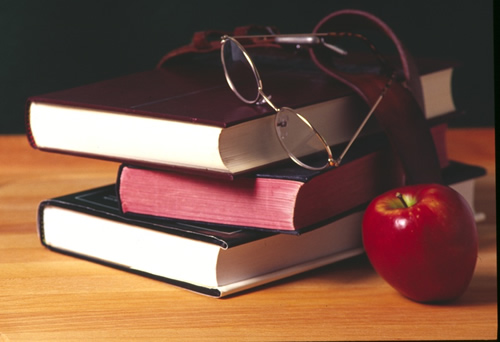•# Welcome To My Website

Mr. Burnett
Room 222

This is my 5th year teaching at Broadview Middle School.  Blended learning in conjunction with PBL (Problem Based/Project Based Learning)will be used in our classroom. My goal is to provide a real-world hands on learning environment for our students that is both informative and fun! Center activities are part of blended learning and will also be incorporated. Feel free to ask questions and I will do my best to provide answers. I look forward to working with our students.

During this school year we will cover the following topics:

• Chapter 4: Areas of Polygons
• Big Ideas Math Chapter 4 Lessons 1-4
• Chapter 8: Surface Area and Volume
• Big Ideas Math Chapter 8 Lessons 2 and 3
• Chapter 1: Numerical Expressions and Factors
• Big Ideas Math Chapter 1 Lessons 4,5,6
• Chapter 5: Ratios and Rates
• Big Ideas:  Chapter 5 Lesson 1,2,3,5,6
• Chapter 2: Fractions and Decimals
• Big Ideas:  Chapter 2 Lessons 2-3
• Chapter 1: Numerical Expressions and Factors
• Big Ideas:  Chapter 1 lesson 1
• Chapter 2: Fractions and Decimals
• Big Ideas:  Chapter 2 Lessons 4-6
• Chapter 6: Integers and the Coordinate Plane
• Big Ideas Math Chapter 6 Lesson 1,3,5,2,4
• Chapter 4: Areas of Polygons
• Big Ideas Math Chapter 4 Lesson 4 (Revisited Polygons in Coordinate Plane)
• Chapter 1: Numerical Expressions and Factors
• Big Ideas Ch. 1 Lessons 2,3,5
• Chapter 3: Algebraic Expressions and Properties
• Big Ideas Math Chapter 3 Lesson 1,2,3,4
• Chapter 7: Equations and Inequalities
• Big Ideas Math Ch 7 Lesson 2,3,6,7,1,4,5
• Chapter 8: Surface Area and Volume
• Big Ideas Math Ch 8 Lesson 4
• Chapter 9: Statistical Measures
• Big Ideas Math Chapter 9 Lesson 1,3,4
• Chapter 10: Data Displays
• Big Ideas Math Chapter 10 Lesson 3
• Chapter 9: Statistical Measures
• Big Ideas Math Ch. 9 Lessons 2,3,4,5
• Chapter 10: Data Displays
• Big Ideas Math Chapter 10 Lessons 1,2,3,4